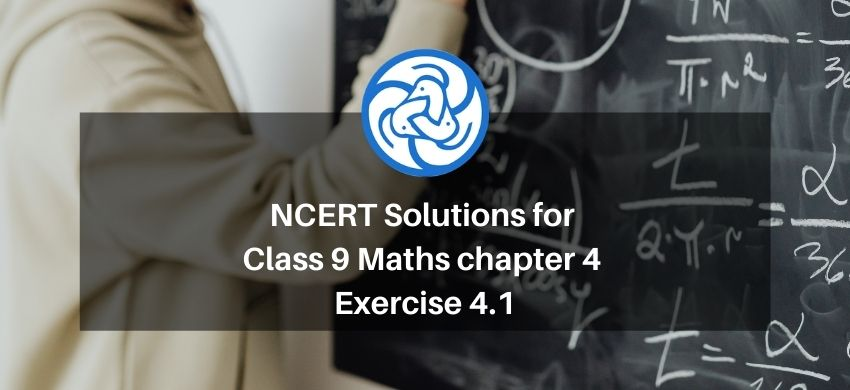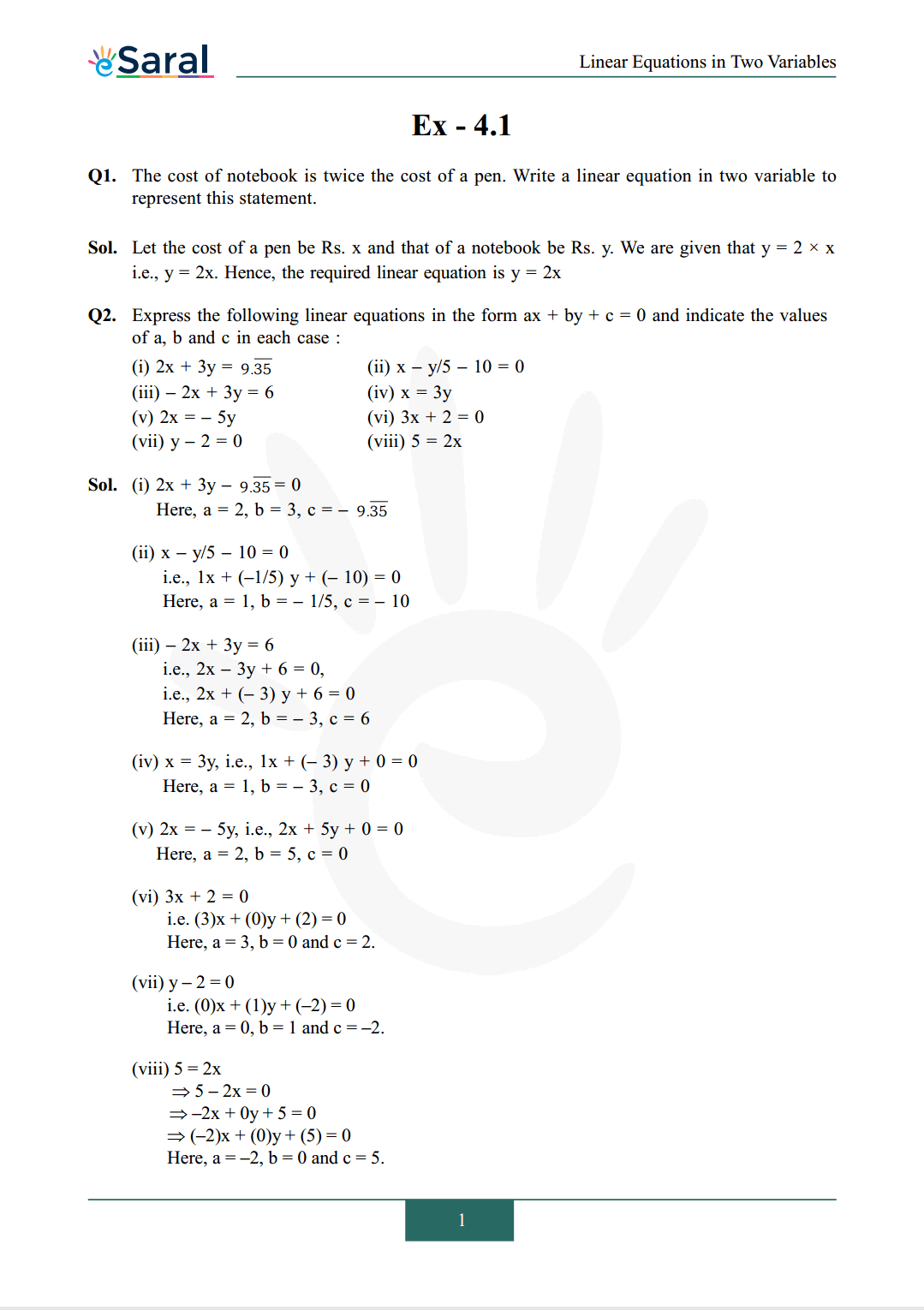Most Affordable JEE | NEET | 8,9,10 Preparation by Kota's Top IITian Doctor Faculties

# NCERT Solutions for Class 9 Maths chapter 4 Exercise 4.1 - Linear Equations in Two Variables`
Hey, are you a class 9 Student and Looking for Ways to Download NCERT Solutions for Class 9 Maths chapter 4 Exercise 4.1? If Yes then you are at the right place.

Here we have listed Class 9 maths chapter 4 exercise 4.1 solutions in PDF that is prepared by Kota’s top IITian’s Faculties by keeping Simplicity in mind.

If you want to score high in your class 9 Maths Exam then it is very important for you to have a good knowledge of all the important topics, so to learn and practice those topics you can use eSaral NCERT Solutions.

So, without wasting more time Let’s start.

### Download The PDF of NCERT Solutions for Class 9 Maths chapter 4 Exercise 4.1 "Linear Equations in Two Variables"#### All Questions of Chapter 4 Exercise 4.1

Once you complete the chapter 4 then you can revise Ex. 4.1 by solving following questions

Q1. The cost of notebook is twice the cost of a pen. Write a linear equation in two variable to represent this statement.

Q2. Express the following linear equations in the form $\mathrm{ax}+\mathrm{by}+\mathrm{c}=0$ and indicate the values of $\mathrm{a}, \mathrm{b}$ and $\mathrm{c}$ in each case :
(i) $2 \mathrm{x}+3 \mathrm{y}=9 . \overline{35}$
(ii) $x-y / 5-10=0$
(iii) $-2 x+3 y=6$
(iv) $x=3 y$
(v) $2 x=-5 y$
(vi) $3 \mathrm{x}+2=0$
(vii) $\mathrm{y}-2=0$
(viii) $5=2 \mathrm{x}$

If you have any Confusion related to NCERT Solutions for Class 9 Maths chapter 4 Exercise 4.1 then feel free to ask in the comments section down below.

To watch Free Learning Videos on Class 9 by Kota’s top Faculties Install the eSaral App